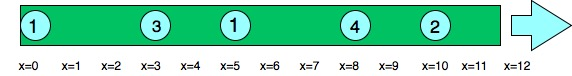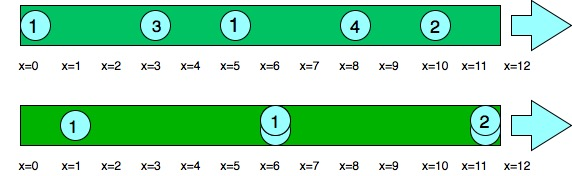# Water drop problem

Consider a pipe of length L. The pipe has N water droplets at N different positions within it. Each water droplet is moving towards the end of the pipe(x=L) at different rates. When a water droplet mixes with another water droplet, it assumes the speed of the water droplet it is mixing with. Determine the no of droplets that come out of the end of the pipe.
Refer to the figure below:
Numbers on circles indicates speed of water dropletsExamples:

```Input: length = 12, position = [10, 8, 0, 5, 3],
speed = [2, 4, 1, 1, 3]
Output: 3
Explanation:
Droplets starting at x=10 and x=8 become a droplet,
meeting each other at x=12 at time =1 sec.
The droplet starting at 0 doesn't mix with any
other droplet, so it is a drop by itself.
Droplets starting at x=5 and x=3 become a single
drop, mixing with each other at x=6 at time = 1 sec.
Note that no other droplets meet these drops before
the end of the pipe, so the answer is 3.
Refer to the figure below
Numbers on circles indicates speed of water droplets.```

## Recommended: Please try your approach on {IDE} first, before moving on to the solution.

Approach:
This problem uses greedy technique.
A drop will mix with another drop if two conditions are met:
1. If the drop is faster than the drop it is mixing with
2. If the position of the faster drop is behind the slower drop.
We use an array of pairs to store the position and the time that ith drop would take to reach the end of the pipe. Then we sort the array according to the position of the drops. Now we have a fair idea of which drops lie behind which drops and their respective time taken to reach the end.More time means less speed and less time means more speed. Now all the drops before a slower drop will mix with it. And all the drops after the slower drop with mix with the next slower drop and so on.
For example if the times to reach the end are- 12, 3, 7, 8, 1 (sorted according to positions)
0th drop is slowest, it won’t mix with the next drop
1st drop is faster than the 2nd drop so they will mix and 2nd drop is faster than 3rd drop so all three will mix together. They cannot mix with the 4th drop because that is faster.
So we use a stack to maintain the local maxima of the times.
No of local maximal + residue(drops after last local maxima) = total no of drops

 `#include ` `using` `namespace` `std; ` `int` `drops(``int` `length, ``int` `position[], ``int` `speed[], ``int` `n) ` `{     ` `    ``// stores position and time taken by a single ` `    ``// drop to reach the end as a pair ` `    ``vector > m(n); ` ` `  `    ``int` `i; ` `    ``for` `(i = 0; i < n; i++) { ` ` `  `        ``// calculates distance needs to be  ` `        ``// covered by the ith drop ` `        ``int` `p = length - position[i];  ` ` `  `        ``// inserts initial position of the  ` `        ``// ith drop to the pair   ` `        ``m[i].first = position[i];  ` ` `  `        ``// inserts time taken by ith drop to reach ` `        ``// the end to the pair ` `        ``m[i].second = p * 1.0 / speed[i];  ` `    ``} ` ` `  `    ``// sorts the pair according to increasing  ` `    ``// order of their positions ` `    ``sort(m.begin(), m.end());  ` `    ``int` `k = 0; ``// counter for no of final drops ` ` `  `    ``// stack to maintain the next slower drop  ` `    ``// which might coalesce with the current drop ` `    ``stack<``double``> s;  ` ` `  `    ``// we traverse the array demo right to left  ` `    ``// to determine the slower drop ` `    ``for` `(i = n - 1; i >= 0; i--) ` `    ``{ ` `        ``if` `(s.empty()) { ` `            ``s.push(m[i].second); ` `        ``} ` ` `  `        ``// checks for next slower drop ` `        ``if` `(m[i].second > s.top()) ` `        ``{ ` `            ``s.pop(); ` `            ``k++; ` `            ``s.push(m[i].second); ` `        ``} ` `    ``} ` ` `  `    ``// calculating residual drops in the pipe ` `    ``if` `(!s.empty())  ` `    ``{ ` `        ``s.pop(); ` `        ``k++; ` `    ``} ` `    ``return` `k; ` `} ` ` `  `// driver function ` `int` `main()  ` `{ ` `    ``int` `length = 12; ``// length of pipe ` `    ``int` `position[] = { 10, 8, 0, 5, 3 }; ``// position of droplets ` `    ``int` `speed[] = { 2, 4, 1, 1, 3 }; ``// speed of each droplets ` `    ``int` `n = ``sizeof``(speed)/``sizeof``(speed); ` `    ``cout << drops(length, position, speed, n); ` `    ``return` `0; ` `} `

Output:

```3
```

My Personal Notes arrow_drop_upCheck out this Author's contributed articles.

If you like GeeksforGeeks and would like to contribute, you can also write an article using contribute.geeksforgeeks.org or mail your article to contribute@geeksforgeeks.org. See your article appearing on the GeeksforGeeks main page and help other Geeks.

Please Improve this article if you find anything incorrect by clicking on the "Improve Article" button below.